• English
• Account
• API
• Integrations
• Enterprise
• Resources
Troubleshooting common Airtable formula errors
• 07 Dec 2023
• Dark
Light
• PDF

# Troubleshooting common Airtable formula errors

• Dark
Light
• PDF

Article Summary

You may encounter errors when building formulas or using one of Airtable's computed fields. These error codes include `#ERROR`, `invalid formula`, `NaN`, `circular reference`, and `infinity`. Below are more details about these common errors and how to fix them.

## Introduction

 Plan availability All plan types Platform(s) Web/Browser, Mac app, and Windows app Related reading Getting started with formulas

## Invalid formulas or #ERRORs in Airtable

The #ERROR or invalid formula error warnings point to a number of potential problems (a catch-all for any errors other than those listed in the following sections). We currently don't display any more information about the source of the error, so you'll need to troubleshoot to find the source.

Some of the typical mistakes you should look for when encountering #ERROR are:

• Mis-typed or non-existent field names, or mistyped functions

• Missing {curly brackets} around field references

• Missing or misplaced (parenthesis) around separate functions in your formula

• Inclusion of smart or curly quotes (e.g. ‘value’) , rather than single quotes (e.g. 'value')

• A null value (e.g. referencing a date field that has no value)

• A missing value after an equals sign

As an example, look over the formula below. You'll notice that it has all of the elements described above; correctly typed field names, correctly placed curly brackets around field references, and sets of parentheses around each function in the formula (hint: there should always be an even number of parentheses).

``````IF(
{Test Score} > 80,
"Exceeds expectations ✅",

IF(
{Test Score} < 80,
"Does not meet expectations ❌",
"Meets expectations..."
)
)
``````

## NaN errors in Airtable

Standing for not a number, the `NaN` error appears when you attempt to divide zero by zero. To fix the error, the most simple approach is to run a valid formula that results in a definable number (e.g. anything but 0 / 0). However, you may want to conditionally run a different calculation for instances when two fields both have a zero value.

For example, if you're tracking progress towards a goal, you would use the formula `{Actual} / {Goal}` to display progress towards that goal as a percent. However, because formulas apply to every record in a table, `NaN` shows for any records where there are zero values in both fields.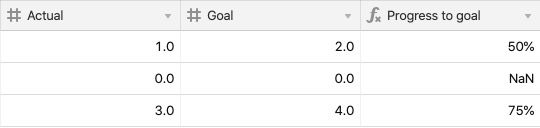To account for instances where there are zero values, you can adjust your formula to this: `IF({Actual} > 0, {Actual} / {Goal},"0%")`. With this adjusted formula, a different value is displayed when there are two zero values.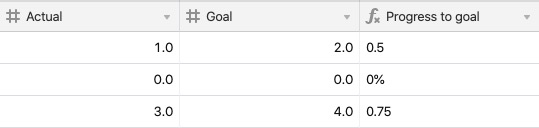You may also see this error when referencing a date field that doesn't have a value. To fix the error in those fields, add in a valid date. To account for empty cells using a formula, use an IF statement similar to the one above. In the example below, an `IF(AND())` statement is used to only calculate the difference between two dates if both date fields have a value, preventing any NaN errors.

``````IF(
AND(
{Start},
{End}
),
DATETIME_DIFF({End},{Start},'days'),
BLANK()
)
``````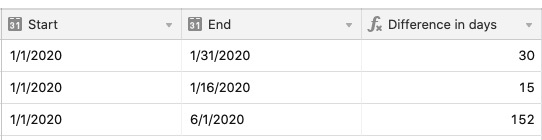## Circular reference errors in Airtable

A circular reference error occurs when one field is trying to calculate off a second field, while the second field is trying to calculate off the first. It doesn't know where to start and creates a loop that can't be completed.

In the example below, the {Name} field is referencing itself, causing a circular reference error.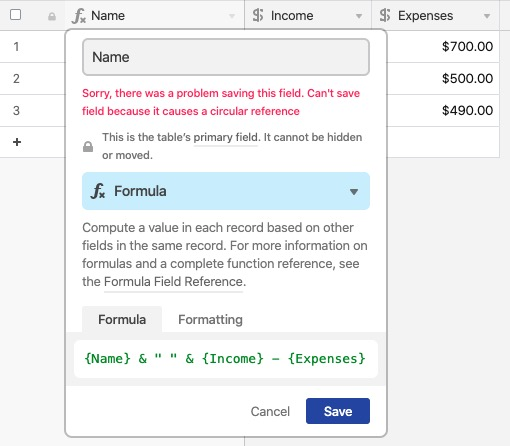To uncover the circular reference, take a closer look at what other fields your formula is referencing -- it's likely referencing another formula field, which in turn is referencing (perhaps indirectly, through yet another formula field!) the original formula field (the one throwing the "circular reference" error when trying to save the formula.

That's why it's circular -- the value of this other field can't be known without knowing the value of the field you're trying to save, which in turn relies on the value of that other field.

## Infinity errors in Airtable

The infinity error occurs when you divide a number by zero (which equals infinity). Since there isn't a way to represent this as a number, you'll need to adjust your formula to divide by a number other than zero.

As an example, an engineering team is working on tracking a quota of bugs addressed by each teammate. In the screenshot below, you'll see an infinity error when dividing `{Completed Issues}` by `{Bug Quota}`.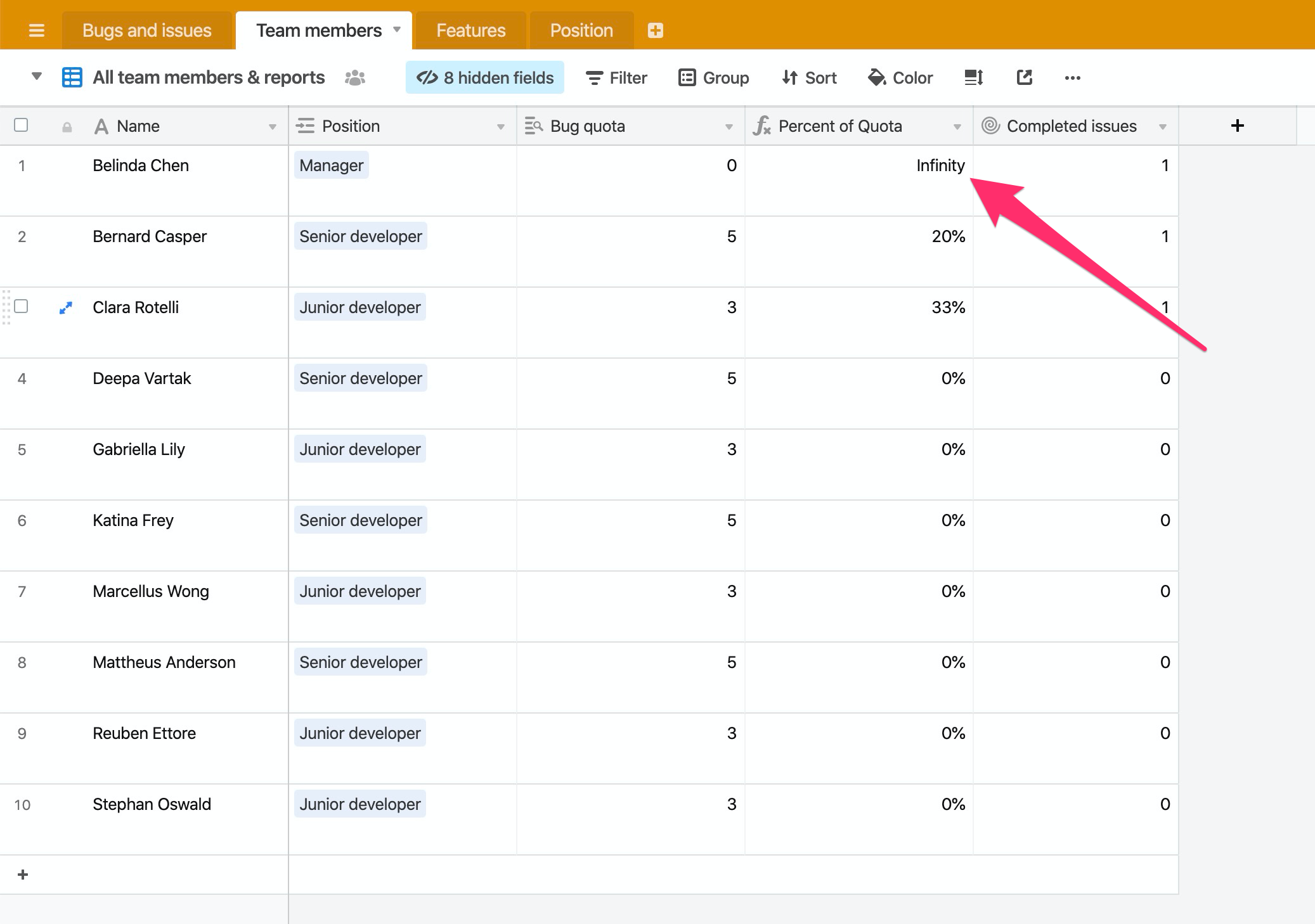By adding in a short conditional statement (using an IF statement), we can only run the division formula when there is a value greater than `0` in the `{Bug Quota}` field. The formula used below is: `IF({Bug quota}=0,BLANK(),{Completed issues}/{Bug quota})`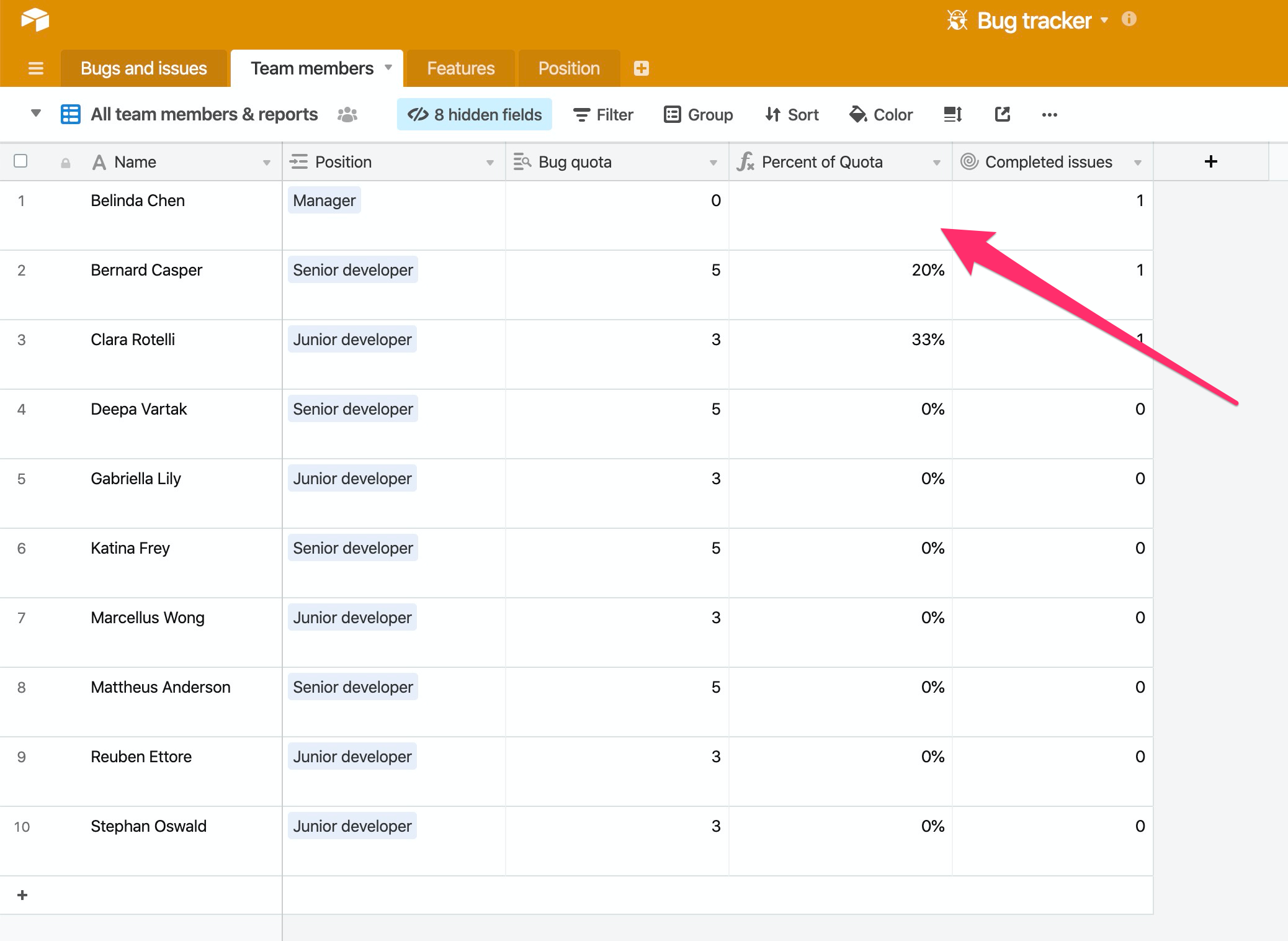## Formatting issues in Airtable

You may want to format the output of a formula as a number, or a date, but are receiving this message: "Your result type is not a number or a date. Formatting options are currently only available if your result type is a number or a date."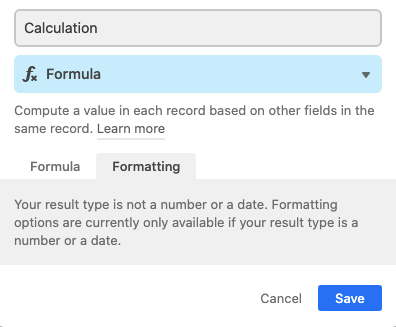This message displays in the formatting tab of a formula field because the result of the formula is not a number or date - that means that there aren't any formatting options to apply to the field. An example of why that could occur is if your formula has the ability to output both numbers and text (or numbers and a date, a date and text, etc).

In the example below, a formula is used to either produce the total cost for an event based on attendees and cost, which would be a number. But, if data for either of the referenced fields is missing, it outputs a message in plain text. The result of this formula could be both numbers and text, so Airtable can't apply any formatting to the field as a whole.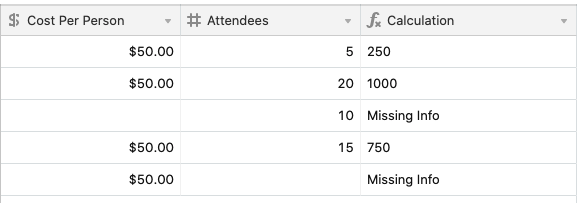To get around this the formula used will need to be adjusted to only output a certain type of date (text, numeric values, or a date). See the before and after formula used for the calculation above.

### Before

``````IF(
AND(
{Cost Per Person},
Attendees
),
{Cost Per Person}*Attendees,
"Missing Info"
)
``````

### After

``````IF(
AND(
{Cost Per Person},
Attendees
),
{Cost Per Person}*Attendees
)
``````

Now that the formula has been adjusted to only output numeric values, the formatting tab displays several formatting options.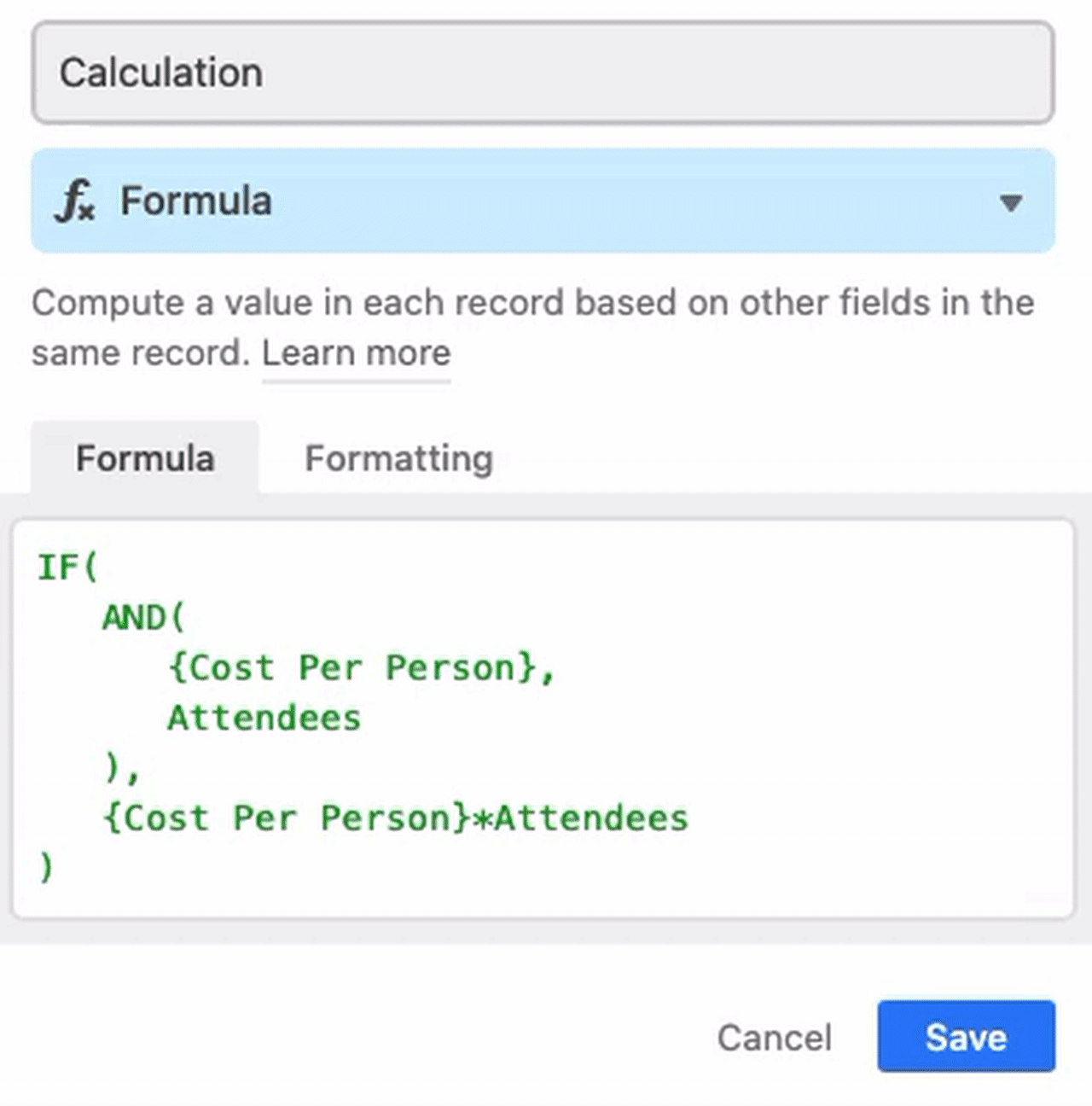## Other errors in Airtable

### Curly quotes

The formula field only recognizes straight quotes, formatted as `""`or `''`. We don't currently accept curly or other stylized quotation marks. These can often be imported if you paste in text or a formula from another source, so be on the lookout for them if you receive a formula error.

### Spaces between functions and opening parenthesis

The formula box does not allow spaces after the opening parenthesis of any function name. For example, this formula would not be accepted due to the space after `IF`:

``````IF ( {Number Field} = 1, "Yes", "No" )
``````

While this similar formula would be accepted:

``````IF( {Number Field} = 1, "Yes", "No" )
``````